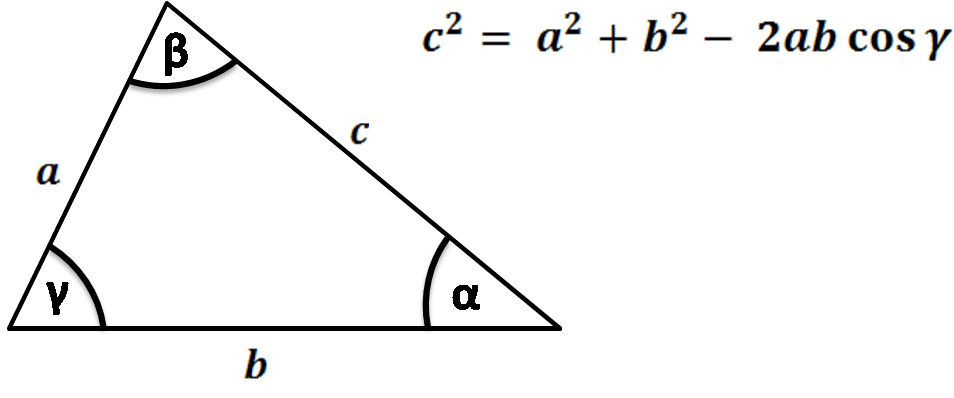# Denz Focus Controller

From the horse’s mouth:The device projects two images at angles $\alpha$ and $\beta$. If you aren’t at the intersection of those two images, they will shift left and right to each other because $\alpha, \beta \neq 0$. If you color code the two images or otherwise distinguish them, you get both the magnitude of the focusing error and the sign. This is basically how phase detect autofocus worked before they crammed it on the sensor.

Assume for a second that $\alpha \equiv -\beta \equiv \tfrac{1}{2} \gamma$; the two little projection lenses are symmetric about some line. We know from geometry that if you draw a line between the two projectors and connect their marginal rays, you have a triangle. We have the law of cosines: $$c^2 = a^2 + b^2 - 2ab \cos\gamma$$From our symmetry argument, $a=b$ and $c$ is the center to center separation of the two projection lenses. We let wolfram alpha do a little simplification: $$a = \frac{c}{\sqrt{2 - 2\cos\gamma}}$$ Suppose $c$ is 35 mm, and the angle between the two projectors is 30°, then $a$ is 67.6 mm. We just need to project $a$ along the normal to get $z$, the distance from the projection lenses to the focus: $$z = a \cos\alpha$$ and see $z$ is 65.3 mm. Now assume we’ve designed this well, or made a prototype and saw the alternative wasn’t too bright, and the projection lenses sit a little inside from the flange - you know, so you don’t smack them into your camera when you put this tool on the body - and that the distance we measure from, the principal plane, is not at the back surface of the lens. Geometric optics can teach you why that is, but just trust me if you don’t want to do a lot of math.

Then doing all those things, this 67 mm might look more like, say, 52 mm which is the FFD of PL mount.

So at this point we know how to design the Denz, but what happens when there’s a coverglass? Well, all that amounts to is a path length adjustment through a window of glass. Snell says (post pic of shirt here)

$$n \sin\theta = n’ \sin\theta’$$ Inside the glass of thickness $t$, the ray will travel a distance of:

$$t’ = \frac{t}{\cos\theta’}$$ You can manipulate these to make an awful expression that turns into something “prettier”:

$$t’ = \frac{t}{\sqrt{1 - \left( \frac{n\sin\theta}{n’}\right)^2}}$$

No matter the <stuff> between the projector and its focus, the optical distance will be 65.3 mm. In air, that’s the same thing as the physical distance. This piece of glass offsets that. Suppose $t$ is 2.25mm, $\theta$ has to be the same as $\alpha$, and $n$ is something around 1.5, then $t'$ is 2.38 mm.

There’s a common saying in optical design that windows make room for themselves, and this is why. If you designed your Denz for a coverglass of 2mm and then used it with a camera that has a 7 mm thick coverglass, it would have a systematic error of 300 microns. If you’re doing differential measurements (comparing cameras, say), there is no upset, it works just as well as it did for the 2mm coverglass.

If you aren’t reconfiguring between a RED and a Canon and have the more reasonable difference of 1.5 and 2 mm, then your systematic error is 30 μm.

If you want to have the device measure for a range of flange focal distances, all you need to do is add a control that pushes the two projectors closer together and further apart without altering the angle between them. If you want an exercise, you can figure out the functional form that has to take:

$$\frac{\partial z}{\partial c} = \space ?$$

And then what geometry you would use to do that, and what cam profile you can cut in a tube of metal to make it happen.